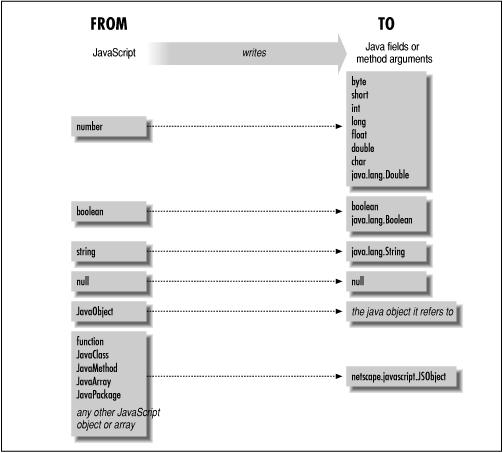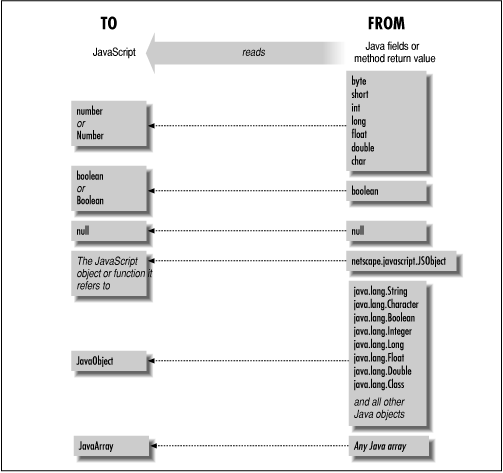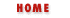#Chapter 19LiveConnect: JavaScript and Java## 19.3 LiveConnect Data Conversion

Java is a strongly typed language with a relatively large number of data types. JavaScript is an untyped language with a relatively small number of types. Because of these major structural differences in the two languages, one of the central responsibilities of LiveConnect is data conversion. When JavaScript sets a Java class or instance field or passes an argument to a Java method, a JavaScript value must be converted to an equivalent Java value. And when JavaScript reads a Java class or instance field or obtains the return value of Java method, that Java value must be converted into a compatible JavaScript value.

 In addition, data conversion must also happen when Java reads or writes a JavaScript field or invokes a JavaScript method. These conversions are done differently, however, and will be described later in the chapter when we explain how to use JavaScript from Java. For now, we're only considering the data conversion that happens when JavaScript code interacts with Java, not the other way around.

Figure 19.2 and Figure 19.3 illustrate how data conversion is performed when JavaScript writes Java values and when it reads them.

#### Figure 19.2: Data conversions performed when JavaScript writes Java values#### Figure 19.3: Data conversions performed when JavaScript reads Java valuesNotice the following points about the data conversions illustrated in Figure 19.2.

• JavaScript numbers can be converted to any of the primitive Java numeric types. The actual conversion performed will depend, of course, on the type of the Java field being set or method argument being passed. Note that you can lose precision doing this, for example, when you pass a large number to a Java field of type short, or when you pass a floating-point value to a Java integral type.

• JavaScript numbers can also be converted to instances of the java class java.lang.Double, but not to instances of related classes such as java.lang.Integer or java.lang.Float.

• JavaScript does not have any representation for character data, so JavaScript numbers may also be converted to the Java primitive char type.

• A JavaObject in JavaScript is "unwrapped" when passed to Java, and is converted to the Java object it represents. Note, however, that JavaClass objects in JavaScript are not converted to Java instances of java.lang.Class, as might be expected.

Also notice these points about the conversions illustrated in Figure 19.3.

• Since JavaScript does not have a type for character data, the Java primitive char type is converted to a JavaScript number, and not a string, as might be expected.

• The figure shows that Java numbers are returned either as primitive JavaScript numbers or as a JavaScript Number object. Similarly, Java boolean values are returned as primitive JavaScript Booleans or as JavaScript Boolean objects. Which is returned depends on whether the value read is a Java field or the return value of a Java method. The discrepancy will be explained in a subsection later in the chapter.

• Java instances of java.lang.Double, java.lang.Integer, and similar classes are not converted to JavaScript numbers. Like all Java objects, they are converted to JavaObject objects in JavaScript.

• Java strings are instances of java.lang.String, so like other Java objects they are converted to JavaObject objects rather than to actual JavaScript strings.

• Any type of Java array is converted to a JavaArray object in JavaScript. Note, however, that Java instances of java.lang.Class are not converted to a JavaClass object--like other Java objects, they are converted to a JavaObject.

### Wrapper Objects

In addition to the note above, there is a very important concept that must be made clear in order for you to fully understand Figure 19.2 and Figure 19.3. This is the idea of "wrapper" objects. While conversions between most JavaScript and Java primitive types are possible, conversions between object types are not, in general, possible. This is why LiveConnect defines the JavaObject object in JavaScript--it represents a Java object that cannot be directly converted to a JavaScript object. In a sense, a JavaObject is a JavaScript "wrapper" around a Java object. When JavaScript reads a Java value (a field or the return value of a method), Java objects are "wrapped" and JavaScript sees a JavaObject.

A similar thing happens when JavaScript writes a JavaScript object into a Java field or passes a JavaScript object to a Java method. There is no way to convert the JavaScript object to a Java object, so the object gets wrapped. Just as the JavaScript wrapper for a Java object is a JavaObject, the Java wrapper for a JavaScript object is the Java class netscape.javascript.JSObject.

It gets interesting when these wrapper objects are passed back. If JavaScript writes a JavaObject into a Java field or passes it to Java method, then LiveConnect first "unwraps" the object, converting the JavaObject back into the Java object that it represents. And similarly, if JavaScript reads a Java field or gets the return value of a Java method that is an instance of netscape.javascript.JSObject, then that JSObject is also unwrapped to reveal and return the original JavaScript object.

### Java Field Values versus Method Return Values

In Navigator 3.0, LiveConnect returns slightly different data types when a value is read from a Java field than it does when the same value is read as the return value of a Java method. Figure 19.3 shows that all Java primitive numeric types and instances of java.lang.Double are returned as primitive JavaScript numbers or as Number objects. When the numeric return value of a method is read, it is returned as a primitive JavaScript number. But when a numeric value is read from a field, it is returned as a Number object.

Recall that Number objects in JavaScript behave almost the same, but not exactly, as primitive JavaScript numbers. One important difference is that Number objects, like all JavaScript objects, use the + operator for string concatenation rather than addition. So code like the following can yield unexpected results:

```var r = new java.awt.Rectangle(0,0,5,5);
var w = r.width;         // This is a Number object, not a primitive number.
var new_w = w + 1;       // Oops!  new_w is now "51", not 6, as expected.
```
To work around this problem, you can explicitly call the valueOf() method to convert a Number object to its corresponding numeric value. For example:

```var r = new java.awt.Rectangle(0,0,5,5);
var w = r.width.valueOf();  // Now we've got a primitive number.
var new_w = w + 1;          // This time, new_w is 6, as desired.
```
You can also force a Number object to a primitive number by using it in a numeric context (but not the + operator) by subtracting zero, for example. So in the above example we could also have done this:

```var w = r.width - 0;        // Now we've got a primitive number.
```

The same discrepancy occurs when Java primitive Boolean values and instances of java.lang.Boolean are read from Java fields--they are returned as JavaScript Boolean objects even though the same Java value would have been returned as a primitive Boolean value if it had been the return value of a method. You can work around this with the valueOf() method, as above.

Finally, when Java objects are read from Java fields (but not when they are read as the return value of a Java method), the returned value behaves in all respects like a JavaObject, except that passing it to the getClass() function fails with an error: "getClass expects a Java object argument". To work around this problem, to obtain a JavaObject object that getClass() recognizes as such, you can use code like the following:

```var o = java.lang.System.out;       // This should be a JavaObject
var c = getClass(o);                // ...but this causes an error.
var p = new Object(o);              // This is the workaround
var c = getClass(p);                // ...this works now.
```

The fact that values are returned differently when read from a field than when read as method return values is not exactly a bug in LiveConnect; it is more of a misfeature, and it is one that the designers of LiveConnect may not be able to correct in future versions of Navigator. It stems from a subtle incompatibility between Java and JavaScript. In Java methods are not data types as they are in JavaScript, so it is perfectly legal to define a method that has the same name as a field. JavaScript, however, allows us to treat methods, including Java methods, as variables that we can manipulate, and so it is not possible to use the same name for a JavaScript property and a method.

We run into a problem when we try to use a Java class has a field and a method by the same name. Suppose that a JavaObject o refers to an instance of such a class, and the name shared by the field and the method is f. Then the JavaScript expression o.f is ambiguous; JavaScript does not know whether we are referring to the method or the field. Consider this code:

```var ambiguous = o.f;     // Is it a JavaMethod or JavaObject?
// It depends on how we use it in the future!
ambiguous();             // Hmm...we must have meant the method.
s += ambiguous;          // In this case, we must have meant the field.
```
The variable ambiguous really can't have a value until it is used in a context that makes it clear what value it is supposed to have. The way this ambiguity is resolved is that ambiguous is implemented as an internal object of a type known as a JavaSlot. Only when it is clear what context the "slot" is being used in is this value converted to the appropriate type.

Notice that this ambiguity only arises when reading Java fields; there is no possibility of it when reading the return values of Java methods. Thus the differences the way values are read arises from the JavaSlot conversion process when Java field values are read.LiveConnect Data TypesJavaScript Conversion of JavaObjects